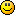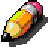You are not logged in.

## #1 2009-07-23 04:40:25

BlueChip
Moderator

### [DONE] Modulo arithmetic is expensive

#### Code:

`a % b`

...is a very expensive operation as it is essentially a complex division

For value of b where b is a power of 2 (Eg 2, 4, 8, 16, 32, etc) the same effect can be achieved by a very cheap AND (&) operation

#### Code:

`a % b  ==  a & (b - 1)`

Therefore

#### Code:

`    offset = (((y >> 2)<<4)*tex->w) + ((x >> 2)<<6) + (((y%4 << 2) + x%4 ) << 1); // Fuckin equation found by NoNameNo ;)`

as used by GRRLIB_GetPixelFromtexImg and GRRLIB_SetPixelTotexImg ...can be rewritten as:

#### Code:

`    offset = (((y >> 2)<<4)*tex->w) + ((x >> 2)<<6) + (((y&3 << 2) + x&3 ) << 1); // Fuckin equation found by NoNameNo ;)`

Also

#### Code:

```    u8 r, g, b, a;
a=*(truc+offset);
r=*(truc+offset+1);
g=*(truc+offset+32);
b=*(truc+offset+33);
return ((r<<24) | (g<<16) | (b<<8) | a);```

...is (sort of) readable code, but again, if the compiler does not realise the cpu has enough registers to perform the operation and furthermore go on to optimise the code, it'll probably store all those values in RAM for a while.

This can be replaced by a slightly less friendly, but more efficient:

#### Code:

`  return (*(truc+offset) <<24) | (*(truc+offset+1) <<16) | (*(truc+offset+32) <<8) | *(truc+offset+33) ;`

These optimisations will help many functions (including the new composition function) run fasterBC

I can be found on efnet, freenode, msn, gtalk, aim, ychat & icq ...PM me for details

Offline

## #2 2009-07-23 18:06:34

Crayon### Re: [DONE] Modulo arithmetic is expensive

Thanks, for the information. On a forum I found those assembly lines to represent each function. I don't know it's on which architectures, but it's interesting.

For input & 0xFF:

#### Code:

```   1. movzx   eax, BYTE PTR [esp + 4]
2. ret```

For input % 256:

#### Code:

```   1.     mov eax, DWORD PTR [esp + 4]
2.     and eax, -2147483393
3.     jge .B2.4
4.     sub eax, 1
5.     or  eax, -256
6.     inc eax
7. .B2.4:
8.     ret```

Changes as been done in revision 103.

Offline